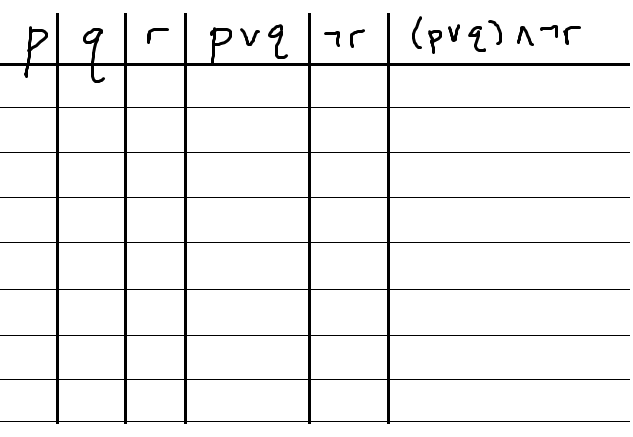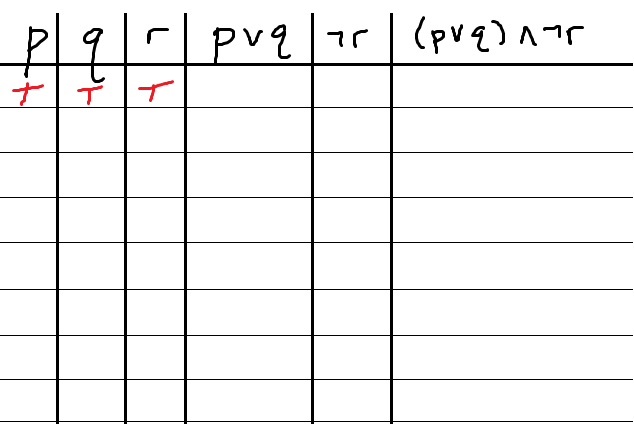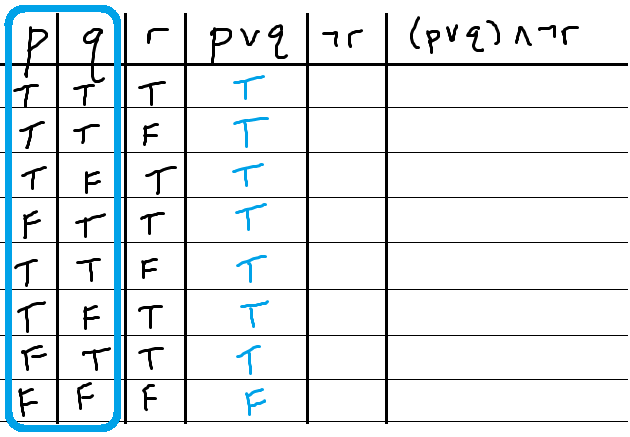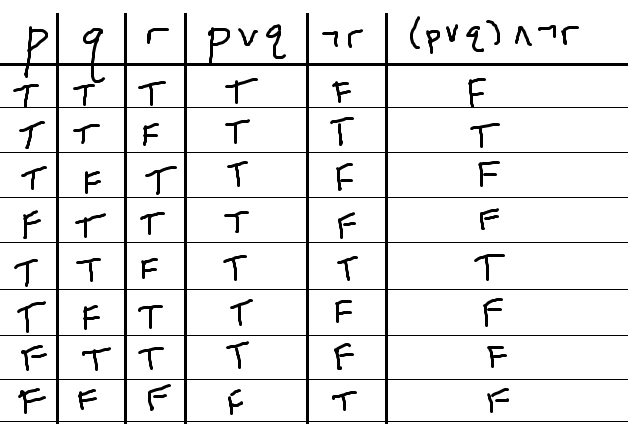﻿ Analyzing compound propositions with truth tables - MathBootCamps

# Analyzing compound propositions with truth tables

For compound propositions, a truth table shows under what conditions the compound statement is valid. This is just like basic truth tables for “and”, “or”, negation, etc but now we have a statement that utilizes more than one of these logical operators. To see how to approach these, we will carefully work through an example.

## Example – compound proposition

Construct the truth table for the following compound proposition.

$$\left(p \vee q\right) \wedge \neg r$$

### Step 1: Set up your table

You need to have your table so that each component of the compound statement is represented, as well as the entire statement itself. In this case, that would be p, q, and r, as well as:

• $$p \vee q$$
• $$\neg r$$
• $$\left(p \vee q\right) \wedge \neg r$$

Thus the initial table set up would be:The order of the columns is not actually all that important. Ideally, you would put p,q,r next to each other so that you can be sure to write ever combination of possible truth values for them without missing any. After that, it is a good strategy to put pieces you will work with together. For instance, the 4th and 5th columns are the statements we combine to make the last column.

### Step 2: Write out all the possible combinations of truth values for each individual proposition

In this case, we want to think of all the combinations of truth values for p, q, and r. It is important to be very systematic here so that you don’t miss any possibilities. Here is one way to think about it:

All statements could be true.Two could be true, which is the same as one false. The false proposition could be any of the three.Two could be false, which is the same as one true. The true proposition could be any of the three.All three could be false.Getting all the combinations covered is very important. A mistake here will throw off the entire table. One quick check, is that by the multiplication rule, there should be $$2 \times 2 \times 2 = 8$$ possibilities. We have 8 rows, so that’s a good sign! (although it is still possible we mixed something up somewhere, so always double check).

### Step 3: Complete the rest of the table using the basic properties or “and”, “or”, and negation

To complete this table, we need to apply the rules for “or” with p, q, apply the rule for negation for r, and then apply the rule for “and” for the last column. If you dont know these rule, you can review them here: Truth tables for and, or, and negation.

Finding $$p \vee q$$. The “or” statement is true in all cases except when both p,q are false.Finding $$\neg r$$. This will have the opposite truth value of r.Finding $$\left(p \vee q\right) \wedge \neg r$$. This is an “and” statement for two of our columns. “And” is only true when both statements are true.This is it – the final answer. A truth table that allows you to see all the conditions under which the compound proposition is true or false.In addition to simply being a tool for understanding the compound statement on its own, this could now be used to determine whether or not a system is consistent and, if we were to add some columns to this, whether or not another statement is equivalent to this one. You can also use the truth tables of compound propositions to determine whether or not you are looking at a tautology, contradiction, or neither.

## Summary

These types of truth tables don’t have to be difficult as long as you are very systematic with your approach. The issues come up when you lose track of which columns you are working with or when you miss a possible combination of truth values in the very beginning of your set up.